# Math Challenge - February 2019

• Challenge
• Featured
Gold Member
Time for our new winter challenge! This time our challenge has also two Computer Science related questions and a separate section with five High School math problems. Enjoy!

Rules:

a)
In order for a solution to count, a full derivation or proof must be given. Answers with no proof will be ignored.
b) It is fine to use nontrivial results without proof as long as you cite them and as long as it is "common knowledge to all mathematicians". Whether the latter is satisfied will be decided on a case-by-case basis.
c) If you have seen the problem before and remember the solution, you cannot participate in the solution to that problem.
d) You are allowed to use google, wolframalpha or any other resource. However, you are not allowed to search the question directly. So if the question was to solve an integral, you are allowed to obtain numerical answers from software, you are allowed to search for useful integration techniques, but you cannot type in the integral in wolframalpha to see its solution.
e) Mentors, advisors and homework helpers are kindly requested not to post solutions, not even in spoiler tags, for the challenge problems, until 16th of each month. This gives the opportunity to other people including but not limited to students to feel more comfortable in dealing with / solving the challenge problems.
f) High School problems are intended exclusively for High School students. There is no point to be solved by people with higher education, so members are kindly requested to respect this rule as well.

Questions

Recurrence relations and algorithms (Questions ##1## and ##2##)

In the cases that an algorithm includes a recursive call, its running time can be expressed via a recurrence relation or equivalently via an equality or inequality which gives the general term of a sequence as a function of smaller terms.
The main techniques used for solving recurrence relations are

- Guess: We guess the solution and then by substitution and utilizing mathematical induction, we try to prove that we guessed right. This method is used when we don't seek for an exact solution but for finding an upper bound. In many cases a good guess can be found using the recursion tree.

- Iteration method (a.k.a. expansion or unfolding method or repeated substitution): This is a (usually) laborious method as we repeatedly apply the given recurrence relation till we find an easily solvable form.

- Master method: As its name implies, it is a "recipe" for solving recurrence relations of the form
##T(n) = a T(\frac{n}{b}) + f(n),\space\space\space\space a, b \geq 1, f(n) > 0, \forall n \geq n_0## where ##a, b## are constants, ##f(n)## is an asymptotically positive function and the division ##\frac{n}{b}## can be translated as either ##\lfloor \frac{n}{b} \rfloor## or ##\lceil \frac{n}{b} \rceil##. Such recurrence relations are present in the analysis of algorithms that use the divide-and-conquer technique. In rough lines, the solution of a problem amounts to the recursive solution of ##a## sub-problems, each of size ##
\frac{n}{b}## and the combination of these individual solutions. The function ##f(n)## represents the cost of both the segmentation in ##a## sub-problems and the combination of their respective solutions.

##1##. (solved by @lpetrich ) Find an upper bound for the recurrence relation ##T(n) = 2T(\lfloor \frac{n}{2} \rfloor) + 1## using the guess method in an optimal way. If ##T(1) = 4## find the lower bounds for the values of constants.

##2##. (solved by @lpetrich ) Solve the recurrence relation ##T(n) = 2T(\frac{n}{3}) + n##,##\space\space\space\space T(1) = 1##. Assume that ##n## is a power of ##3##.

##3##. (solved by @lpetrich ) Let ##f(x)=\dfrac{(\cos \varphi - \sqrt{3}\sin \varphi +1)x+2\sqrt{3}\sin \varphi}{x^2}##
and ##g(x)=\dfrac{(\cos \varphi - \sqrt{3}\sin \varphi -1)x+2\sqrt{3}\sin \varphi}{x^2}##. For which values of ##\varphi## are ##f \perp g## in ##L^2([1,\infty))\,?##

##4##. (solved by @lpetrich ) Let ##x## be a real number. Find ##\lim_{n\to\infty}n\left(\left(1+x/n\right)^n-e^x\right).##

##5##. a.) (solved by @QuantumP7 ) Compute the last three digits of ##3^{2405}\,.##
##\space\space\space\space##b.) (open) Show that there is an integer ##a \in \mathbb{Z}## such that ##64959\,|\,(a^2-7)\,.##

##6##. (solved by @Periwinkle ) Let ##f: [0,1] \rightarrow \mathbb{R}## be a continuous function with ##f(0) = f(1)## . Prove that the equation ##f(x) = f(x + \frac{1}{n})## has at least one real root, for all ## n = 1, 2, 3,\dots##

##7##. Let ##\zeta## be a primitive ##7##th root of unity. For how many ##(a_1,\ldots,a_6)\in \{0,1\}^6## is it true that ##\mathbb{Q}(a_1\zeta+\ldots+a_6\zeta^6)=\mathbb{Q}(\zeta)\,?##

##8##. (solved by @Periwinkle ) In a conference there are ##n## persons. Show that there are two persons such that, from the rest ##n - 2## persons, there are at least ##\lfloor \frac{n}{2} \rfloor - 1## persons each of which knows both the aforementioned two persons or else knows no one of them. Suppose that "know someone" is a symmetric relation.

##9##. Let ##A## be a real square matrix. Show that if ##A-I## is nilpotent, then ##A## has a square root.

##10##. If an ##n \times n## matrix ##A## satisfies ##A^2 - 3 A + 2 \mathbb{I} = \mathbb{O}## where ##\mathbb{I}## is the ##n \times n## identity matrix and ##\mathbb{O}## the ##n \times n## zero matrix, prove that for all positive integers ##n \geq 1## holds ##A^{2n} - (2^n + 1)A^n + 2^n \mathbb{I} = \mathbb{O}##.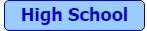##1##. (solved by @Leo Consoli ) A man wants to figure out the length of an escalator, i.e. the number of steps ##[N]## if it was out of order. Since it wasn't out of order, he counted ##60## steps if he walks with the stairs and ##90## steps if he walks in the opposite direction. What is ##[N]?##

##2##. (solved by @Young physicist ) We are looking for a number with eight digits: two of each ## 1,2,3,4##. The ones are separated by one other number, the twos by two, the threes by three, and the fours by four other numbers.

##3##. (solved by @archaic ) Which of you four threw the ball in my window?
##A## says: It was ##E##.
##E## says: It was ##G##.
##F## says: It was not me.
##G## says: ##E## lied
a.) If only one of the four lied, who threw the ball?
b.) If only one person has told the truth, who was the culprit?

##4##. (solved by @Ujjwal Basumatary ) Choose any two but different natural numbers and form their sum, their difference and product. Prove that among these three numbers at least one is divisible by ##3##.

##5##. (solved by @Leo Consoli ) Prove that the remainder in dividing any prime by ##30## is either ##1## or prime again. Is this also true when dividing a prime number by ##60\,?##

#### Attachments

Last edited by a moderator:
•Periwinkle, atyy, Greg Bernhardt and 4 others

## Answers and Replies

High school 2:
41312432

I found that literally by trial and error though, not sure what the proper method is.

The only “ method” I use is that because 4 has to be separated by 4 numbers, the are only 3 possible positions, which I then can trial and error easier.

•atyy
Mentor
2021 Award
High school 2:
41312432

I found that literally by trial and error though, not sure what the proper method is.

The only “ method” I use is that because 4 has to be separated by 4 numbers, the are only 3 possible positions, which I then can trial and error easier.
Yes, conclusions and exclusions are probably the only way here. However, your answer is not complete.

Yes, conclusions and exclusions are probably the only way here. However, your answer is not complete.
More than one answer?

Mentor
2021 Award
Yes.

•YoungPhysicist
Mentor
2021 Award
More than one answer?
Are you still trying to find it? Please do so, for a) it's quite easy and b) an important lesson to learn here!

Are you still trying to find it? Please do so, for a) it's quite easy and b) an important lesson to learn here!
Yeah, I am just playing baseball for the past couple hours. I’ll do that as soon as I finished my game.

Last edited:
•atyy
High school 2 second attempt:
23421314 is also correct (wait, isn’t that the first one reversed? Is that the lesson?)

•atyy
Mentor
2021 Award
High school 2 second attempt:
23421314 is also correct (wait, isn’t that the first one reversed? Is that the lesson?)
Yes, it is, at least one half of it. The other half to learn is: do not make hidden assumptions or be satisfied too early! In this case the assumption, that one solution already does it, because the way to find it has left apparently no other possibilities. That's a mathematical standard: There are always two parts: Does a solution exist at all? If so, is it unique?

When it comes to solution spaces, always look out for symmetries! They can make life a lot easier, e.g. to restrict a proof on one case as others are symmetric. They can also show the structure of solutions. O.k. maybe not so much in this simple case, but in general. Symmetries are of extraordinary importance in mathematics as well as in physics. So always look out for symmetries.

•maline, atyy and YoungPhysicist
High school 3a:
E lied.

Process:
If A lied, then E lied as well since what G said is the truth. Contradiction.

If E lied, all other statements suggest E is the one throwing the ball.

If F lied, then the one throwing the ball is F, making A and E lying as well. Contradiction.

If G lied, than G is the one throwing the ball, then A lied as well. Contradiction.

archaic
High school 3
a) E lied and is the culprit, else there would be contradictions.
b) G told the truth and F is the culprit, contradictions otherwise.

•atyy and fresh_42
Gold Member
3
##<f, g> = 0## with ##<f, g> = \int_1^\infty fgdx##. Since we are in ℝ this operator is bilinear and so:
$$<f, g> = (cos \phi - \sqrt{3} sin \phi + 1)(cos \phi - \sqrt{3} sin \phi - 1) \left< \frac 1 x, \frac 1 x \right> + (2\sqrt{3} sin \phi) (cos \phi - \sqrt{3} sin \phi + 1) \left< \frac 1 x, \frac 1 {x^2} \right> + (2\sqrt{3} sin \phi) (cos \phi - \sqrt{3} sin \phi - 1) \left< \frac 1 {x^2}, \frac 1 x \right> + (2\sqrt{3} sin \phi)^2 \left< \frac 1 {x^2}, \frac 1 {x^2} \right> = 0$$
Evaluating:
$$\left< \frac 1 x, \frac 1 x \right> = 1$$
$$\left< \frac 1 {x^2}, \frac 1 x \right> = 2$$
$$\left< \frac 1 {x^2}, \frac 1 {x^2} \right> = 3$$
Here I was lazy and I used Mathematica to simplify the above expression, but if that is against the rule please delete this and I will do the calculation by hand. I got:
$$2 sin \phi (3 \sqrt{3} cos\phi+ 7 sin\phi) = 0$$
So ##\phi = k \pi## with ##k = 0, 1, 2....## and ##\phi = -arctan \left( \frac {3 \sqrt{3}} 7 \right) + \frac {k \pi} 2## with ##k = 0, 1, 2....##

Mentor
2021 Award
3
##<f, g> = 0## with ##<f, g> = \int_1^\infty fgdx##. Since we are in ℝ this operator is bilinear and so:
$$<f, g> = (cos \phi - \sqrt{3} sin \phi + 1)(cos \phi - \sqrt{3} sin \phi - 1) \left< \frac 1 x, \frac 1 x \right> + (2\sqrt{3} sin \phi) (cos \phi - \sqrt{3} sin \phi + 1) \left< \frac 1 x, \frac 1 {x^2} \right> + (2\sqrt{3} sin \phi) (cos \phi - \sqrt{3} sin \phi - 1) \left< \frac 1 {x^2}, \frac 1 x \right> + (2\sqrt{3} sin \phi)^2 \left< \frac 1 {x^2}, \frac 1 {x^2} \right> = 0$$
Evaluating:
$$\left< \frac 1 x, \frac 1 x \right> = 1$$
$$\left< \frac 1 {x^2}, \frac 1 x \right> = 2$$
$$\left< \frac 1 {x^2}, \frac 1 {x^2} \right> = 3$$
Here I was lazy and I used Mathematica to simplify the above expression, but if that is against the rule please delete this and I will do the calculation by hand. I got:
$$2 sin \phi (3 \sqrt{3} cos\phi+ 7 sin\phi) = 0$$
So ##\phi = k \pi## with ##k = 0, 1, 2....## and ##\phi = -arctan \left( \frac {3 \sqrt{3}} 7 \right) + \frac {k \pi} 2## with ##k = 0, 1, 2....##
I have a different result, but I cannot see whether I did a mistake, which is possible as I constructed the problem myself, or you did, since you neither gave a complete proof nor does your formula fit on the page.

And yes, a complete solution is required, no hidden computer algorithms.

Edit: I have checked my result again, so I think you made a mistake.

Last edited:
Gold Member
your formula fit on the page.
Yes it does... Scroll to the right and you will see the whole equation.

no hidden computer algorithms.
I just used the command
Code:
Simplfy[ ---copy-paste that long equations---]
Does that mean I have to do it by hand ?

I have a different result
I checked the result I got putting it in the starting functions and I got the expected result, but I could have made some copy/paste mistake myself

Mentor
2021 Award
I checked the result I got putting it in the starting functions and I got the expected result, but I could have made some copy/paste mistake myself
I double checked it again. Have you checked for ##\pi/6## which is as far as I can see not in your list?

Gold Member
I double checked it again. Have you checked for π/6π/6\pi/6 which is as far as I can see not in your list?
You are right, ##\pi/6## is a solution I have overlooked. The strange thing is that if I put ##\pi/6## in the equation I wrote in post #12 it doesn't yield zero... but the integral is actually zero. Is there something wrong in my answer ? Are the other results correct ? I maybe be just hitting some wrong keys on the keyboard because it's 3 am hereTomorrow I'll try again. I'm really sorry for all the trouble.

Mentor
2021 Award
Is there something wrong in my answer ?
I think there has to be. If I made no mistake, then there is an elegant solution of it with not many easy, i.e. polynomial integrals.

QuantumP7
4. Let ##x## be a real number. Find ##\lim_{n \rightarrow \infty}n((1+\frac {x}{n})^{n} - e^{x})##.

##\lim_{n \rightarrow \infty}n((1+\frac {x}{n})^{n} - e^{x}) = \left( \lim_{n \rightarrow \infty}n * \lim_{n \rightarrow \infty}(1+\frac {x}{n})^{n} \right) - \left( \lim_{n \rightarrow \infty}n * \lim_{n \rightarrow \infty} e^{x} \right)##

##= \left( \lim_{n \rightarrow \infty}n * e^{x} \right) - \left( \lim_{n \rightarrow \infty}n * e^{x} \right) ##

##=0##

Gold Member
##\lim_{n \rightarrow \infty}n((1+\frac {x}{n})^{n} - e^{x}) = \left( \lim_{n \rightarrow \infty}n * \lim_{n \rightarrow \infty}(1+\frac {x}{n})^{n} \right) - \left( \lim_{n \rightarrow \infty}n * \lim_{n \rightarrow \infty} e^{x} \right)##

This is not right, as neither of the terms on your righthand side exist.

Leo Consoli
Hi, here is my attempt for high school problem 1:
Being N the number of steps when the escalator is not working, s the size of a step of the escalator and u the speed of the escalator.
In the first situation when the person is going up:
s is the relative velocity to the escalator, and (u+s) is the velocity to the ground, so the movement equation is: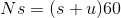In the second situation: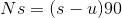Dividing both by s: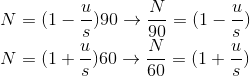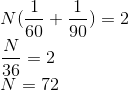#### Attachments

QuantumP7
4. Let x be a real number. Find ## \lim_{n \rightarrow \infty} n \left( \left( 1 + \frac{x}{n} \right) ^{n} - e^{x} \right)##

##\lim_{n \rightarrow \infty} ln \left( n \left( \left(1 + \frac{x}{n} \right) ^{n} - e^{x} \right) \right)##

## = \lim_{n \rightarrow \infty} \left( ln(n) + ln \left( \left(1 + \frac{x}{n} \right) ^{n} - e^{x} \right) \right) ##
## = \lim_{n \rightarrow \infty} \left( ln(n) + ln \left(1 + \frac{x}{n} \right) ^{n} - ln \left( e^{x} \right) \right) ##
## = \lim_{n \rightarrow \infty} \left( ln(n) \right) + \lim_{n \rightarrow \infty} \left( ln \left(1 + \frac{x}{n} \right) ^{n} - ln \left( e^{x} \right) \right) ##
## = \lim_{n \rightarrow \infty} \left( ln(n) \right) + \lim_{n \rightarrow \infty} \left( ln \left(1 + \frac{x}{n} \right) ^{n} \right) - \lim_{n \rightarrow \infty} \left( ln \left( e^{x} \right) \right) ##
## = \lim_{n \rightarrow \infty} \left( ln(n) \right) + \left( x \right) - \lim_{n \rightarrow \infty} \left( x \right) ##
## = \lim_{n \rightarrow \infty} \left( ln(n) \right) + \left( x \right) - \left( x \right) ##
## = \lim_{n \rightarrow \infty} \left( ln(n) \right) ##
##= \infty ##

Gold Member
## = \lim_{n \rightarrow \infty} \left( ln(n) + ln \left( \left(1 + \frac{x}{n} \right) ^{n} - e^{x} \right) \right) ##
## = \lim_{n \rightarrow \infty} \left( ln(n) + ln \left(1 + \frac{x}{n} \right) ^{n} - ln \left( e^{x} \right) \right) ##

This step is not right, as $\ln(A-B)$ is not the same as $\ln(A)-\ln(B)$.

QuantumP7
This step is not right, as $\ln(A-B)$ is not the same as $\ln(A)-\ln(B)$.

Aw. You're right.

4. Let x be a real number. Find ## \lim_{n \rightarrow \infty} n \left( \left( 1 + \frac{x}{n} \right) ^{n} - e^{x} \right)##

## \lim_{n \rightarrow \infty} n \left( \left( 1 + \frac{x}{n} \right) ^{n} - e^{x} \right)##
##= \left( \lim_{n \rightarrow \infty} n \right) \left( \lim_{n \rightarrow \infty} \left( \left( 1 + \frac{x}{n} \right) ^{n} - e^{x} \right) \right)##
##= \left( \lim_{n \rightarrow \infty} n \right) \left( \lim_{n \rightarrow \infty} \left( 1 + \frac{x}{n} \right) ^{n} - \lim_{n \rightarrow \infty} \left( e^{x} \right) \right) ##
##= \left( \lim_{n \rightarrow \infty} n \right) \left( e^{x} - e^{x} \right) ##
##= \left( \lim_{n \rightarrow \infty} n \right) \left( 0 \right) ##
##= 0##

Am I getting closer? *hopeful smile*

Gold Member
Aw. You're right.

4. Let x be a real number. Find ## \lim_{n \rightarrow \infty} n \left( \left( 1 + \frac{x}{n} \right) ^{n} - e^{x} \right)##

## \lim_{n \rightarrow \infty} n \left( \left( 1 + \frac{x}{n} \right) ^{n} - e^{x} \right)##
##= \left( \lim_{n \rightarrow \infty} n \right) \left( \lim_{n \rightarrow \infty} \left( \left( 1 + \frac{x}{n} \right) ^{n} - e^{x} \right) \right)##
##= \left( \lim_{n \rightarrow \infty} n \right) \left( \lim_{n \rightarrow \infty} \left( 1 + \frac{x}{n} \right) ^{n} - \lim_{n \rightarrow \infty} \left( e^{x} \right) \right) ##
##= \left( \lim_{n \rightarrow \infty} n \right) \left( e^{x} - e^{x} \right) ##
##= \left( \lim_{n \rightarrow \infty} n \right) \left( 0 \right) ##
##= 0##

Am I getting closer? *hopeful smile*

You can't do this, because $0\cdot\infty$ is an indeterminate form and so doesn't always evaluate to zero. This is rather like claiming that $$1=\lim_{x\to\infty} 1=\lim_{x\to\infty} \left(x\cdot\frac{1}{x}\right)=\left(\lim_{x\to\infty} x\right)\left(\lim_{x\to\infty}\frac{1}{x}\right)=\left(\lim_{x\to\infty} x\right)\cdot 0=0.$$

For what it's worth, the answer is not zero or infinity. It depends on $x$.

Gold Member
I have a question regarding #2.

What does "Solve the recurrence relation," mean. That is, what kind of presentation would be a solution?

Gold Member
I have a question regarding #2.

What does "Solve the recurrence relation," mean. That is, what kind of presentation would be a solution?

Questions ##1## and ##2## are about recurrence relations and algorithms. So,- as a recurrence relation can be used to express the running time of an algorithm, by solving a recurrence relation we can find what ##T(n)## says about ##O(n)## and / or ##\Theta (n)## depending on the case at hand.

Now, for ##2##, I ask for solving the specific recurrence relation - you can check the spoiler for a brief summary of the methods used if needed, which means finding a form that is more general including variable(s) for which, applying the right condition(s), you can reach a conclusion about the ##O(n)## (or maybe ##\Theta (n)##) of the algorithm whose running time is expressed by the specific recurrence relation.

Gold Member
Questions 1 and 2 are about recurrence relations and algorithms.
Now, for 2, I ask for solving the specific recurrence relation - you can check the spoiler for a brief summary of the methods used if needed, which means finding a form that is more general including variable(s) for which, applying the right condition(s), you can reach a conclusion about the O(n) (or maybe Θ(n)) of the algorithm whose running time is expressed by the specific recurrence relation.

Hi QQ:

Thank you for your response to my post.

I must confess that I had no expectation that the problem statement involved (1) any algorithms, (2) the behavior of O(n) (or maybe Θ(n)), or (3) running time.
My experience with the O(n) notation is limited to its use with an infinite sum to represent the order of magnitude of the sum of remaining terms in the sum following some specific terms. I do not see what it has to do with the recursion. I also have never seen before the Θ(n) notation.

I did scan the thread quickly and failed to find a spoiler for problem #2. I will now make a more careful perusal.

The spoiler you were referring to was the one included in the OP following the rules. I read through it and found no definition of what is means to "solve" a recursion. Please post a definition of the meaning of "the solution of a recursion".

Also, I am unfamiliar with the notation involving a pair of brackets in the shape of an "L" and its mirror image. Please also post an explanation of this notation.

Regards,
Buzz

Last edited:
Gold Member
I must confess that I had no expectation that the problem statement involved (1) any algorithms, (2) the behavior of O(n) (or maybe Θ(n)), or (3) running time.

The problem statement does not speak explicitly about algorithms but the problem is under the title

Recurrence relations and algorithms (Questions ##1## and ##2##)

so it is evidently about recurrence relations as used in the context of algorithms, so we're talking about the running time of an algorithm - that's the ##T(n)## in the recurrence relation(s), and so we have to do with ##O(n)## and ##\Theta(n)##.

My experience with the O(n) notation is limited to its use with an infinite sum to represent the order of magnitude of the sum of remaining terms in the sum following some specific terms. I do not see what it has to do with the recursion. I also have never seen before the Θ(n) notation.

You can take a look at Wikipedia's Big O Notation.

The ##T(n)## in the given recurrence relation(s) is the running time of an algorithm. By solving the recurrence relation and by the appropriate substitutions after that, you can find bound(s) i.e. ##O(n)##, ##\Theta(n)## etc. I hope that this explains what has ##O(n)## to do with the recursion (expressed via a recurrence relation). As for the ##\Theta(n)## notation take a look at this fsu.edu page for instance.

The spoiler you were referring to was the one included in the OP following the rules. I read through it and found no definition of what is means to "solve" a recursion. Please post a definition of the meaning of "the solution of a recursion".

Yes, that's the spoiler I meant. What I talk about there is methods about solving recurrence relations. As I say there,

In the cases that an algorithm includes a recursive call, its running time can be expressed via a recurrence relation or equivalently via an equality or inequality which gives the general term of a sequence as a function of smaller terms.

Now, about solving a recurrence relation, I give the methods used but (obviously) I can not explain here the whole thing from scratch. You can take a look at this cmu.edu page for instance and also google it for much more.

Also, I am unfamiliar with the notation involving a pair of brackets in the shape of an "L" and its mirror image. Please also post an explanation of this notation.

I think that it is widely known - if I am mistaken about this I apologize in advance, that this is the floor function.

Last edited:
•Buzz Bloom
Gold Member
Now, about solving a recurrence relation, I give the methods used but (obviously) I can not explain here the whole thing from scratch. You can take a look at this cmu.edu page for instance and also google it for much more.
Hi QQ:

Thank you very much for answering my questions.

I took a look at the cmu.edu page you cited, and the 30 page document looks somewhat daunting. It will take some time for me to grasp the relationship between (1) the solution being the estimate of how much time a recursive algorithm takes to execute a calculation given the value of an argument., and (2) solving a recursive function which is somehow related to such an algorithm.

Perhaps you can help me gain an insight by advising me regarding a simple recursive function. For this example I have chosen the
Fibonacci numbers as a recursive function.

F(0) = F(1) = 1
For n>1, F(n) = F(n-1) + F(n-2)

What is the solution of this recursive series?

Regards,
Buzz

Gold Member
Re #4.
I have now completed my work on a second solution.
I apologize for not using LaTex, but the reference I have does not e.g. help me with finite summations.
I have fixed the first error @Infrared pointed out to me in his post #42, and a second error involving a sum that should be a product. I will be working on the other issues he included for a while.

I found that I had made an error regarding the number of factors (1-1/n)(1-2/n)... . There should be only k-1 factors rather than k+1 factors. I have edited the math to reflect correcting this error.
The problem is to find
S(x) = limn→∞ n((1+x/n)n−ex).​
Using the Binomial Theorem gives:
(1+x/n)n = Σ{k=0,n} C(n,k) (x/n)k
where
C(n,k) = n!/(k! (n-k)!) ,​
and
C(n,k)/nk = (1/k!)[(1-1/n)(1-2/n)...(1-(n-k+1)/n]
= (1/k!) [ 1 - (1/n)Σ{j=1,k-1}j + O(1/n2)]
= (1/k!) [ 1 - (1/n) k(k-1)/2 + O(1/n2)]
= 1/k! - (1/k!)(1/n) k(k-1)/2 + O(1/n2)]
Now break S(x) into three parts.
S(x) = S1(x) + S2(x) + S3(x)

S1(x) = limn→∞ n [ Σ{k=0,n} xk/k! - ex ]
= n [ Σ{k=0,∞} xk/k! - ex ] = 0

S2(x) = - limn→∞ n Σ{k=0,n} (1/n) (k(k-1)/2) xk/k!
= - Σ{k=0,∞} (k2-k)/2 xk/k!

S3(x) = limn→∞ n O(1/n2) = 0​

Therefore the solution is
S(x) = S2(x)
= (1/2) (-x2 + x) ex

Last edited:
Gold Member
What is the solution of this recursive series?

Although I think that you can find it easily on the net, in any case, the explicit solution to the Fibonacci recurrence is ##F_n = \frac{1}{\sqrt{5}}(\frac{1 + \sqrt{5}}{2})^n - \frac{1}{\sqrt{5}}(\frac{1 - \sqrt{5}}{2})^n## known as Binet's formula.

QuantumP7
5. a) Find the last 3 digits of ##3^{2405}##

##3^{2405} = \left( 3^{5} \right) ^{481} = \left( 3^{5} \right) ^{400+80+1}##
The last 3 digits of ##3^{2405}## will then be ## \left(3^{5} \right)^{1} = 243##.

Mentor
2021 Award
5. a) Find the last 3 digits of ##3^{2405}##

##3^{2405} = \left( 3^{5} \right) ^{481} = \left( 3^{5} \right) ^{400+80+1}##
The last 3 digits of ##3^{2405}## will then be ## \left(3^{5} \right)^{1} = 243##.
Why?

QuantumP7
Why?

##3^{2405} = 3^{5+2400} = (3^{5})(3^{2400})##
##3^{400} \equiv 1(mod 1000)## so ##3^{2400} \equiv 1(mod 1000)##
Then, ##3^{2405} = (3^{5})(3^{2400}) \equiv (243*1)(mod 1000)##, so that the last 3 digits of ##3^{2405}## are 243.

Last edited:
QuantumP7
5.(b) Show that there is an integer ##a \in \mathbb{Z}## such that 64959| ## (a^{2} - 7)##